# Integration Applications in Calculus Chapter Exam

Exam Instructions:

Choose your answers to the questions and click 'Next' to see the next set of questions. You can skip questions if you would like and come back to them later with the yellow "Go To First Skipped Question" button. When you have completed the practice exam, a green submit button will appear. Click it to see your results. Good luck!

Answered 0 of 25

### Page 1

#### Question 1 1. Given the cut-off cone pictured, with h = x+3 and the region extending from x=2 to x=4, what is the integral you will need to solve to find the volume of this cut-off cone?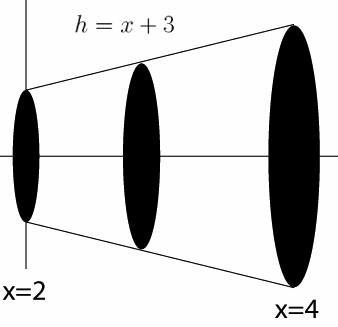#### Question 2 2. What is the volume made by revolving f(x) around the x-axis between x=0 and x=1?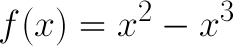#### Question 3 3. What is the volume made by revolving f(x) around the x-axis between x=0 and x=1?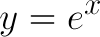#### Question 4 4. Use integration to find the area between the curves g(x) and f(x) between 0 and 1.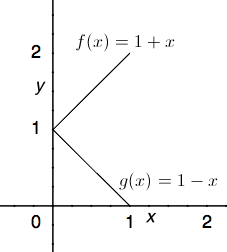#### Question 5 5. What is the volume of the cut-off cone shown?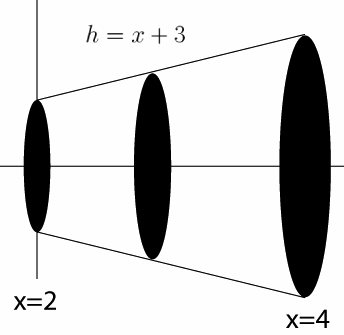### Page 2

#### Question 6 6. What is the equation for the area between f(x) and the x-axis from x=0 to x=2?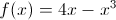#### Question 7 7. If your velocity is given by v(t), and you start at x=4 at t=0, then for what value of t will you be at the position x=20?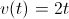#### Question 8 8. Given a velocity v(t) as defined below and a starting position of x=1 at t=0, what is the equation for your position as a function of time?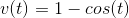#### Question 10 10. Use integration to find the area between the curves h(x) and g(x) between 0 and 2.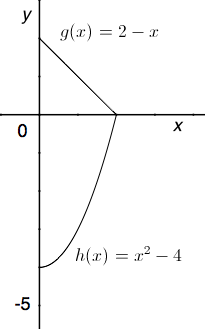### Page 3

#### Question 12 12. Given a velocity of v(t) as defined below, what is the equation for your position as a function of time?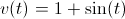#### Question 13 13. Use integration to find the area bounded by f(x), g(x), and h(x).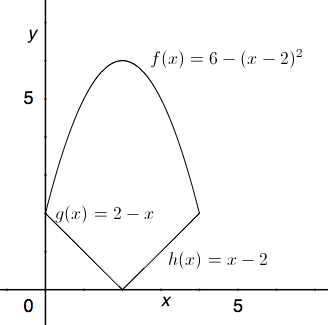#### Question 14 14. What is the equation for the volume of this cone that extends from x=0 to x=A, given that h=x?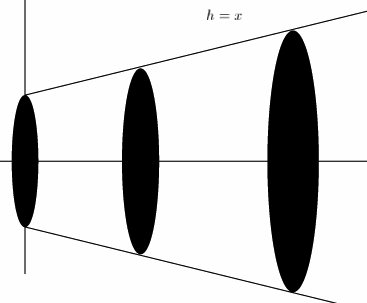#### Question 15 15. What is the area between f(x) and the x-axis from x=0 to x=2?### Page 4

#### Question 16 16. Consider the region bound by the x-axis, the y-axis, and f(x) between 0 and 4. What is the area of this region?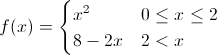#### Question 17 17. What is the volume made by revolving the area between g(x) and f(x) around the x-axis between x=0 and x=1?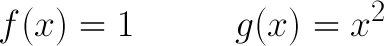#### Question 18 18. What is the area between f(x) and the x-axis from x=-2 to x=0?#### Question 19 19. Consider the region bound by the x-axis, the y-axis, and f(x) between 0 and 4. What is an equation for the area of this region?#### Question 20 20. Use integration to find the area between the curves f(x) and g(x) between 0 and 4.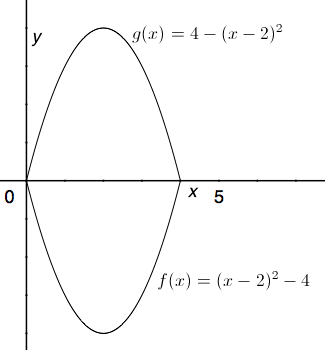### Page 5

#### Question 21 21. Use integration to find the area enclosed by f(x) and g(x).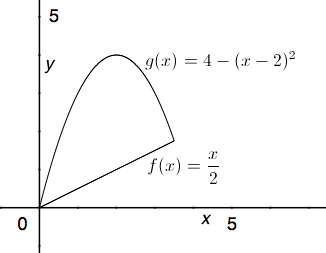#### Question 22 22. What is the equation for the volume of this cone that extends from x=0 to x=A, given that h=x?#### Question 25 25. What is the equation for the volume of this cone that extends from x=0 to x=A, given that h=x?#### Integration Applications in Calculus Chapter Exam Instructions

Choose your answers to the questions and click 'Next' to see the next set of questions. You can skip questions if you would like and come back to them later with the yellow "Go To First Skipped Question" button. When you have completed the practice exam, a green submit button will appear. Click it to see your results. Good luck!

Support